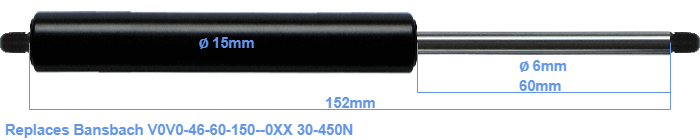# Replacement for Bansbach V0V0-46-60-150–0XX 30-450N

\$26.35

Replacement gas spring for the Bansbach V0V0-46-60-150--0XX 30-450 Newton. Thread: M5. Brand: Stabilus Industry Line.
 Force Choose an option30 Newton40 Newton50 Newton60 Newton80 Newton100 Newton120 Newton140 Newton150 Newton160 Newton180 Newton200 Newton220 Newton240 Newton250 Newton260 Newton280 Newton300 Newton320 Newton340 Newton350 Newton360 Newton380 Newton400 Newton420 Newton440 Newton450 NewtonClearThe bore of the house of this replacement gas spring is 15 mm. The cross section of the rod is 6 mm. The stroke is 60 millimeter long. The total length of this replacement is exactly 152 mm. This is the total distance between the centers of the two mounting parts. The length from thread to thread (exlcuding the mounting parts) is 152 millimeter. This gas spring (V0V0-46-60-150--0XX) has a force of 30-450 Newton. This gas spring can replace a Bansbach gas spring, but is not an orignal Bansbach one. This replacement gas spring is a Stabilus Industry Line gas spring. Nevertheless, the force and dimensions equal.